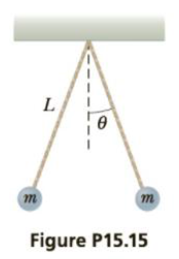Chapter 15, Problem 15P

Chapter
Section
Textbook Problem

Two small metallic spheres, each of mass m = 0.20 g, are suspended as pendulums by light strings from a common point as shown in Figure P15.15. The spheres are given the same electric charge, and it is found that they come to equilibrium when each string is at an angle of θ = 5.0° with the vertical. If each string has length L = 30.0 cm, what is the magnitude of the charge on each sphere?To determine
The magnitude of charge on each sphere.

Explanation

Given info: Mass of the sphere is0.20 g. Length of the string (L) is 30.0 cm.

The free body diagram is given by,

From the above diagram,

Tcos5ο=mg       (I)

Tsin5ο=FC       (II)

• T is the tension.
• m is the mass.
• g is the acceleration due to gravity.
• FC is the Coulomb force.

Divide Equation (II) by (I) and re-arrange.

FC=mgtan5ο       (III)

Formula to calculate the Coulomb force is,

FC=keQ2r2      (IV)

• ke is the Coulomb constant.
• Q is the charge.
• r is the distance of separation.

The distance of separation is,

r=2Lsin5ο=2(30.0cm)sin5ο=5.23cm

Substitute Equation (IV) in (III) and re-arrange to get Q

Q=rmgtan5οke

Substitute 8

Still sussing out bartleby?

Check out a sample textbook solution.

See a sample solution

The Solution to Your Study Problems

Bartleby provides explanations to thousands of textbook problems written by our experts, many with advanced degrees!

Get Started

The smallest unit of life is the _____. a. atom b. molecule c. cell d. organism

Biology: The Unity and Diversity of Life (MindTap Course List)

The body's adipose tissue has a virtually infinite capacity to store fats. T F

Nutrition: Concepts and Controversies - Standalone book (MindTap Course List)

Why does nuclear fusion require high temperatures?

Horizons: Exploring the Universe (MindTap Course List)

Define the following terms: a. chromosome b. chromatin

Human Heredity: Principles and Issues (MindTap Course List)

How is evolution by natural selection thought to operate?

Oceanography: An Invitation To Marine Science, Loose-leaf Versin